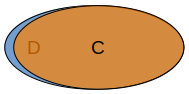# `SubClassOf` vs `EquivalentTo`¶

## Prerequisites¶

This lesson assumes you have basic knowledge wrt ontologies and OWL as explained in:

## `SubClassOf`¶

In this section we explain the semantics of `SubClassOf`, give an example of using `SubClassOf` and provide guidance for when not to use `SubClassOf`.

### Semantics¶

If we have

``````Class: C
SubClassOf: D
Class: D
``````

the semantics of it is given by the following Venn diagram: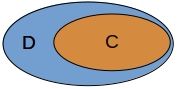Thus, the semantics is given by the subset relationship, stating the `C` is a subset of `D`. This means every individual of `C` is necessarily an individual of `D`, but not every individual of `D` is necessarily an individual of `C`.

### A concrete example¶

``````Class: Dog
SubClassOf: Pet
Class: Pet
``````

which as a Venn diagram will look as follows: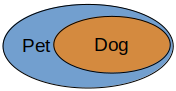### Guidance¶

There are at least 2 scenarios which at first glance may seem like `C SubClassOf D` holds, but it does not hold, or using `C EquivalentTo D` may be a better option.

1. This is typically where `C` has many individuals that are in `D`, but there is at least 1 individual of `C` that is not in `D`. The following Venn diagram is an example. Thus, to check whether you may be dealing with this scenario, you can ask the following question: Is there any individual in `C` that is not in `D`? If 'yes', you are dealing with this scanario and you should not be using `C SubClassOf D`.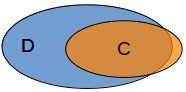1. When you have determined that (1) does not hold, you may deal with the scenario where not only is every individual of `C` in `D`, but also every individual in `D` is in `C`. This means `C` and `D` are equivalent. In the case you rather want to make use of `EquivalentTo`.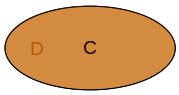## Semantics¶

If we have

``````Class: C
EquivalentTo: D
Class: D
``````

this means the sets `C` and `D` fit perfectly on each other, as shown in the next Venn diagram:Note that `C EquivalentTo D` is shorthand for

``````Class: C
SubClassOf: D
Class: D
SubClassOf: C
``````

though, in general it is better to use `EquivalentTo` rather than the 2 `SubClassOf` axioms when `C` and `D` are equivalent.

### A concrete example¶

We all probably think of humans and persons as the exact same set of individuals.

``````Class: Person
EquivalentTo: Human
Class: Human
``````

and as a Venn diagram: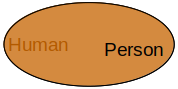### Guidance¶

When do you not want to use `EquivalentTo`?

1. When there is an individual of `C` that is not in `D`.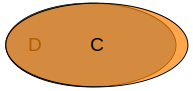1. When there is an individual of `D` that is not in `C`.